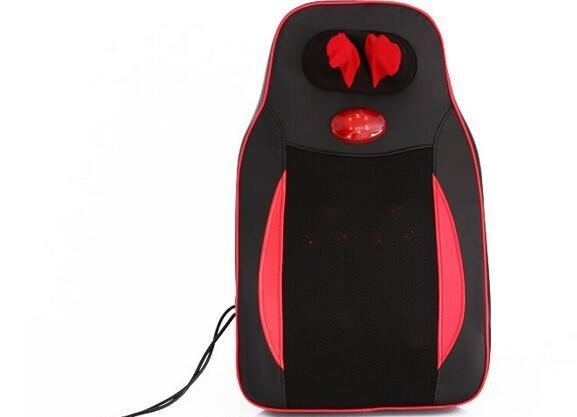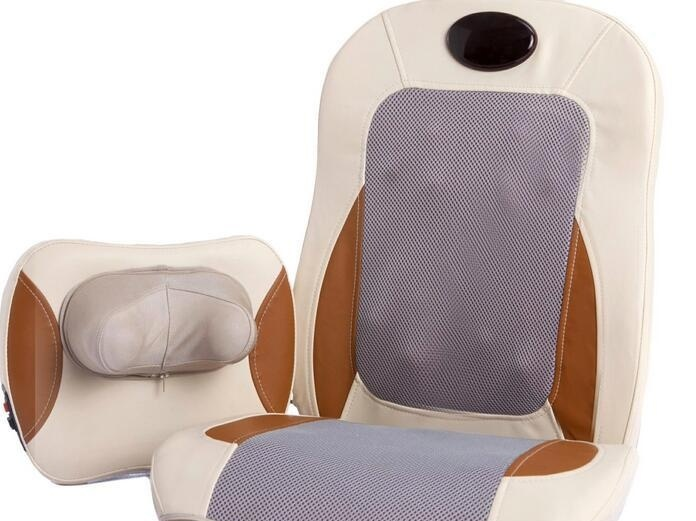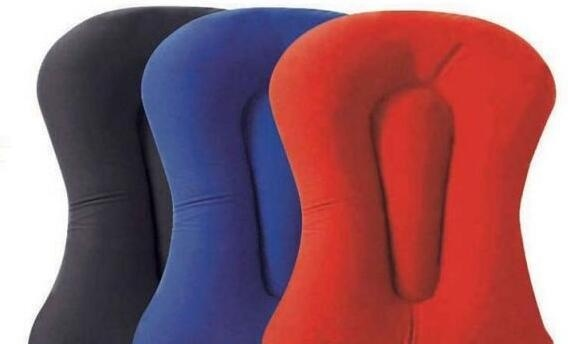|

# 按摩靠垫什么牌子的好 选购技巧介绍

按摩靠垫是一种难得的室内保健类运动器材，非常适合上班族和老年人，眼下马上春节将近不如选择一款送给亲爱的爸爸妈妈尽一份孝心，那么到底按摩靠垫什么牌子的好？选购技巧有哪些呢？下文中将都会为大家讲解到。按摩靠垫品牌推荐－康达

了解完了按摩靠垫的一些基本的知识之后。那么现在我们就来看看这个按摩靠垫哪个牌子好一些。首先，小编要给大家推荐的就是康达这个按摩靠垫品牌。康达按摩靠垫是深圳市焗康维实业有限公司旗下的一个按摩靠垫品牌。这个按摩靠垫公司成立在2003年。康达按摩靠垫这个品牌公司，它是专业的从事设计、研发、生产和销售各类保健器材的公司。公司生产的按摩靠垫产品不仅销往全国各地，这些按摩靠垫产品还会远销到国外市场，这是业界类口碑比较不错的一个按摩靠垫品牌。

按摩靠垫品牌推荐－凯仕乐

了解按摩靠垫产品的朋友们，肯定对凯仕乐这个按摩靠垫品牌并不陌生。凯仕乐它也是专业从事研发、生产和销售各种保健类器材的一个企业。生产按摩靠垫的凯仕乐，它的总部是在美国的纽约。后来，凯仕乐看到了中国市场的强大，于是在后来又和广东省的凯仕乐公司结成了合作伙伴关系。后来广东凯仕乐逐渐的发展和壮大有了自己的一个设计、研发、成产和销售的团队，他们拥有了很多的市级代理商，网络也是覆盖了全国大大小小的城市。至于凯仕乐生产的按摩靠垫产品，有这么强大的一个后盾，那么它的按摩靠垫产品质量也是毋庸置疑的。

按摩靠垫品牌推荐－倍轻松

大家知道，深圳市一个一线城市，在这个城市里，无论什么样的产品，几乎都会在这个充满可能性的城市里找得到。那么在深圳也有一家生产按摩靠垫比较不错的公司，它的名字就是倍轻松。生产按摩靠垫的这家倍轻松公司在1996年的时候成立。公司发展到现在，生产出来的按摩靠垫产品已经非常的成熟，而且广受消费者的认可和追捧。就目前来看。倍轻松的产品项目主要是分为了三个系列，这三个系列分别是头部、眼部、MINI按摩器。按摩靠垫选购技巧介绍

按摩垫选购之看外观

在我们选购按摩垫的时候，选择一款有着良好外观的按摩垫是至关重要的。因为外观的好坏，在某种程度上，会影响我们的心情，进而影响按摩垫的效果。因此，选择一款外观良好的按摩垫，是很重要的哦。

按摩垫选购之看功能

在选购按摩垫的时候，一定要看看按摩垫的相关功能。一般而言，按摩垫的功能其实都是大同小异，差不多的。不过，如果是价格比较贵的按摩垫的话，功能可能会比较齐全和强大。所以，可以根据自己的需要去选择。

按摩垫选购之看功率

有些厂家为了节约成本，会降低按摩垫电机的功率，而卖的价格却和原来的一样。因此，大家在选购按摩垫的时候，一定要特别注意这个问题，千万不要被无良商家欺骗了哦。

按摩垫选购之看服务

在选购按摩垫的时候，一定要选择有着良好售后服务的按摩垫产品。千万不要贪小便宜而忽略了售后服务的选择。毕竟，有了良好的售后服务，我们才能够用得安心。

按摩垫选购之看品牌

在选购按摩垫的时候，最好选择那些牌子响、口碑好的产品。因为毕竟人家的产品是有目共睹的，因此最好选择那些名牌产品。这样的话，不仅质量上有保证，而且在售后服务上，也是很有保障的哦。按摩靠垫有用吗

1、按摩靠垫的用途是非常广泛的，其中最主要的还是有助于缓解人体疲劳。每当人们疲惫的时候，将这个靠垫放在身后，就能够享受到靠垫由上而下的按摩，的确能够很快地放松下来，进而缓解一天忙碌工作之后的疲倦感。

2、同时按摩靠垫据说还能够放松人体肌肉，缓解身体酸痛感，从而促进人们快速的进入睡眠状态。其实这个作用与缓解疲倦有很大的联系，只有当人们放松下来之后，人们的整个身心才会舒适，进而进入睡眠。

3、至于对于有人说这种按摩靠垫能治疗颈椎炎、肩周炎、腰肌劳损、贫血、神经衰弱和失眠等疾病，这种是没有科学依据的，大家不要盲目相信。

`声明：本文由入驻焦点开放平台的作者撰写，除焦点官方账号外，观点仅代表作者本人，不代表焦点立场错误信息举报电话： 400-099-0099，邮箱：jubao@vip.sohu.com，或点此进行意见反馈，或点此进行举报投诉。`A B C D E F G H J K L M N P Q R S T W X Y Z
A - B - C - D - E
• A
• 鞍山
• 安庆
• 安阳
• 安顺
• 安康
• 澳门
• B
• 北京
• 保定
• 包头
• 巴彦淖尔
• 本溪
• 蚌埠
• 亳州
• 滨州
• 北海
• 百色
• 巴中
• 毕节
• 保山
• 宝鸡
• 白银
• 巴州
• C
• 承德
• 沧州
• 长治
• 赤峰
• 朝阳
• 长春
• 常州
• 滁州
• 池州
• 长沙
• 常德
• 郴州
• 潮州
• 崇左
• 重庆
• 成都
• 楚雄
• 昌都
• 慈溪
• 常熟
• D
• 大同
• 大连
• 丹东
• 大庆
• 东营
• 德州
• 东莞
• 德阳
• 达州
• 大理
• 德宏
• 定西
• 儋州
• 东平
• E
• 鄂尔多斯
• 鄂州
• 恩施
F - G - H - I - J
• F
• 抚顺
• 阜新
• 阜阳
• 福州
• 抚州
• 佛山
• 防城港
• G
• 赣州
• 广州
• 桂林
• 贵港
• 广元
• 广安
• 贵阳
• 固原
• H
• 邯郸
• 衡水
• 呼和浩特
• 呼伦贝尔
• 葫芦岛
• 哈尔滨
• 黑河
• 淮安
• 杭州
• 湖州
• 合肥
• 淮南
• 淮北
• 黄山
• 菏泽
• 鹤壁
• 黄石
• 黄冈
• 衡阳
• 怀化
• 惠州
• 河源
• 贺州
• 河池
• 海口
• 红河
• 汉中
• 海东
• I
• J
• 晋中
• 锦州
• 吉林
• 鸡西
• 佳木斯
• 嘉兴
• 金华
• 景德镇
• 九江
• 吉安
• 济南
• 济宁
• 焦作
• 荆门
• 荆州
• 江门
• 揭阳
• 金昌
• 酒泉
• 嘉峪关
K - L - M - N - P
• K
• 开封
• 昆明
• 昆山
• L
• 廊坊
• 临汾
• 辽阳
• 连云港
• 丽水
• 六安
• 龙岩
• 莱芜
• 临沂
• 聊城
• 洛阳
• 漯河
• 娄底
• 柳州
• 来宾
• 泸州
• 乐山
• 六盘水
• 丽江
• 临沧
• 拉萨
• 林芝
• 兰州
• 陇南
• M
• 牡丹江
• 马鞍山
• 茂名
• 梅州
• 绵阳
• 眉山
• N
• 南京
• 南通
• 宁波
• 南平
• 宁德
• 南昌
• 南阳
• 南宁
• 内江
• 南充
• P
• 盘锦
• 莆田
• 平顶山
• 濮阳
• 攀枝花
• 普洱
• 平凉
Q - R - S - T - W
• Q
• 秦皇岛
• 齐齐哈尔
• 衢州
• 泉州
• 青岛
• 清远
• 钦州
• 黔南
• 曲靖
• 庆阳
• R
• 日照
• 日喀则
• S
• 石家庄
• 沈阳
• 双鸭山
• 绥化
• 上海
• 苏州
• 宿迁
• 绍兴
• 宿州
• 三明
• 上饶
• 三门峡
• 商丘
• 十堰
• 随州
• 邵阳
• 韶关
• 深圳
• 汕头
• 汕尾
• 三亚
• 三沙
• 遂宁
• 山南
• 商洛
• 石嘴山
• T
• 天津
• 唐山
• 太原
• 通辽
• 铁岭
• 泰州
• 台州
• 铜陵
• 泰安
• 铜仁
• 铜川
• 天水
• 天门
• W
• 乌海
• 乌兰察布
• 无锡
• 温州
• 芜湖
• 潍坊
• 威海
• 武汉
• 梧州
• 渭南
• 武威
• 吴忠
• 乌鲁木齐
X - Y - Z
• X
• 邢台
• 徐州
• 宣城
• 厦门
• 新乡
• 许昌
• 信阳
• 襄阳
• 孝感
• 咸宁
• 湘潭
• 湘西
• 西双版纳
• 西安
• 咸阳
• 西宁
• 仙桃
• 西昌
• Y
• 运城
• 营口
• 盐城
• 扬州
• 鹰潭
• 宜春
• 烟台
• 宜昌
• 岳阳
• 益阳
• 永州
• 阳江
• 云浮
• 玉林
• 宜宾
• 雅安
• 玉溪
• 延安
• 榆林
• 银川
• Z
• 张家口
• 镇江
• 舟山
• 漳州
• 淄博
• 枣庄
• 郑州
• 周口
• 驻马店
• 株洲
• 张家界
• 珠海
• 湛江
• 肇庆
• 中山
• 自贡
• 资阳
• 遵义
• 昭通
• 张掖
• 中卫

1室1厅1厨1卫1阳台

1
2
3
4
5

0
1
2

1

1

0
1
2
3报名成功，资料已提交审核A B C D E F G H J K L M N P Q R S T W X Y Z
A - B - C - D - E
• A
• 鞍山
• 安庆
• 安阳
• 安顺
• 安康
• 澳门
• B
• 北京
• 保定
• 包头
• 巴彦淖尔
• 本溪
• 蚌埠
• 亳州
• 滨州
• 北海
• 百色
• 巴中
• 毕节
• 保山
• 宝鸡
• 白银
• 巴州
• C
• 承德
• 沧州
• 长治
• 赤峰
• 朝阳
• 长春
• 常州
• 滁州
• 池州
• 长沙
• 常德
• 郴州
• 潮州
• 崇左
• 重庆
• 成都
• 楚雄
• 昌都
• 慈溪
• 常熟
• D
• 大同
• 大连
• 丹东
• 大庆
• 东营
• 德州
• 东莞
• 德阳
• 达州
• 大理
• 德宏
• 定西
• 儋州
• 东平
• E
• 鄂尔多斯
• 鄂州
• 恩施
F - G - H - I - J
• F
• 抚顺
• 阜新
• 阜阳
• 福州
• 抚州
• 佛山
• 防城港
• G
• 赣州
• 广州
• 桂林
• 贵港
• 广元
• 广安
• 贵阳
• 固原
• H
• 邯郸
• 衡水
• 呼和浩特
• 呼伦贝尔
• 葫芦岛
• 哈尔滨
• 黑河
• 淮安
• 杭州
• 湖州
• 合肥
• 淮南
• 淮北
• 黄山
• 菏泽
• 鹤壁
• 黄石
• 黄冈
• 衡阳
• 怀化
• 惠州
• 河源
• 贺州
• 河池
• 海口
• 红河
• 汉中
• 海东
• I
• J
• 晋中
• 锦州
• 吉林
• 鸡西
• 佳木斯
• 嘉兴
• 金华
• 景德镇
• 九江
• 吉安
• 济南
• 济宁
• 焦作
• 荆门
• 荆州
• 江门
• 揭阳
• 金昌
• 酒泉
• 嘉峪关
K - L - M - N - P
• K
• 开封
• 昆明
• 昆山
• L
• 廊坊
• 临汾
• 辽阳
• 连云港
• 丽水
• 六安
• 龙岩
• 莱芜
• 临沂
• 聊城
• 洛阳
• 漯河
• 娄底
• 柳州
• 来宾
• 泸州
• 乐山
• 六盘水
• 丽江
• 临沧
• 拉萨
• 林芝
• 兰州
• 陇南
• M
• 牡丹江
• 马鞍山
• 茂名
• 梅州
• 绵阳
• 眉山
• N
• 南京
• 南通
• 宁波
• 南平
• 宁德
• 南昌
• 南阳
• 南宁
• 内江
• 南充
• P
• 盘锦
• 莆田
• 平顶山
• 濮阳
• 攀枝花
• 普洱
• 平凉
Q - R - S - T - W
• Q
• 秦皇岛
• 齐齐哈尔
• 衢州
• 泉州
• 青岛
• 清远
• 钦州
• 黔南
• 曲靖
• 庆阳
• R
• 日照
• 日喀则
• S
• 石家庄
• 沈阳
• 双鸭山
• 绥化
• 上海
• 苏州
• 宿迁
• 绍兴
• 宿州
• 三明
• 上饶
• 三门峡
• 商丘
• 十堰
• 随州
• 邵阳
• 韶关
• 深圳
• 汕头
• 汕尾
• 三亚
• 三沙
• 遂宁
• 山南
• 商洛
• 石嘴山
• T
• 天津
• 唐山
• 太原
• 通辽
• 铁岭
• 泰州
• 台州
• 铜陵
• 泰安
• 铜仁
• 铜川
• 天水
• 天门
• W
• 乌海
• 乌兰察布
• 无锡
• 温州
• 芜湖
• 潍坊
• 威海
• 武汉
• 梧州
• 渭南
• 武威
• 吴忠
• 乌鲁木齐
X - Y - Z
• X
• 邢台
• 徐州
• 宣城
• 厦门
• 新乡
• 许昌
• 信阳
• 襄阳
• 孝感
• 咸宁
• 湘潭
• 湘西
• 西双版纳
• 西安
• 咸阳
• 西宁
• 仙桃
• 西昌
• Y
• 运城
• 营口
• 盐城
• 扬州
• 鹰潭
• 宜春
• 烟台
• 宜昌
• 岳阳
• 益阳
• 永州
• 阳江
• 云浮
• 玉林
• 宜宾
• 雅安
• 玉溪
• 延安
• 榆林
• 银川
• Z
• 张家口
• 镇江
• 舟山
• 漳州
• 淄博
• 枣庄
• 郑州
• 周口
• 驻马店
• 株洲
• 张家界
• 珠海
• 湛江
• 肇庆
• 中山
• 自贡
• 资阳
• 遵义
• 昭通
• 张掖
• 中卫• 手机• 分享
• 设计
免费设计
• 计算器
装修计算器
• 入驻
合作入驻
• 联系
联系我们
• 置顶
返回顶部